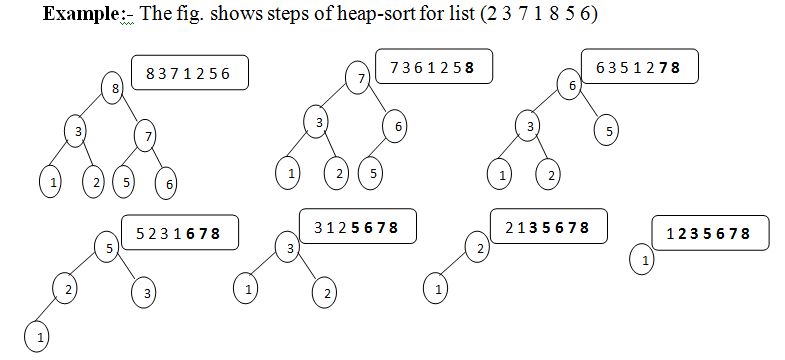# Heap Sort Basic Information

suggest change

Heap sort is a comparison based sorting technique on binary heap data structure. It is similar to selection sort in which we first find the maximum element and put it at the end of the data structure. Then repeat the same process for the remaining items.

Pseudo code for Heap Sort:

``````function heapsort(input, count)
heapify(a,count)
end <- count - 1
while end -> 0 do
swap(a[end],a)
end<-end-1
restore(a, 0, end)

function heapify(a, count)
start <- parent(count - 1)
while start >= 0 do
restore(a, start, count - 1)
start <- start - 1``````

Example of Heap Sort:Auxiliary Space: `O(1)`

Time Complexity: `O(nlogn)`